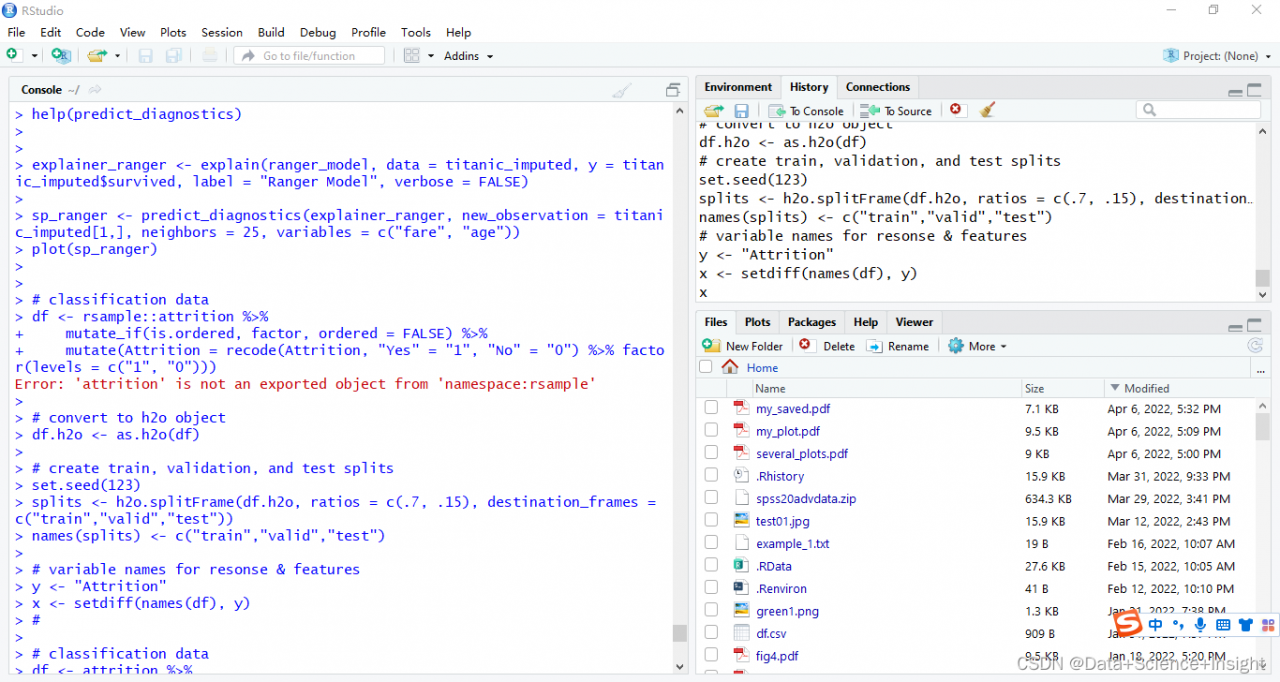# [Solved] RuntimeError: NCCL error in: XXX, unhandled system error, NCCL version 2.7.8

Project scenario:

This problem is encountered in distributed training,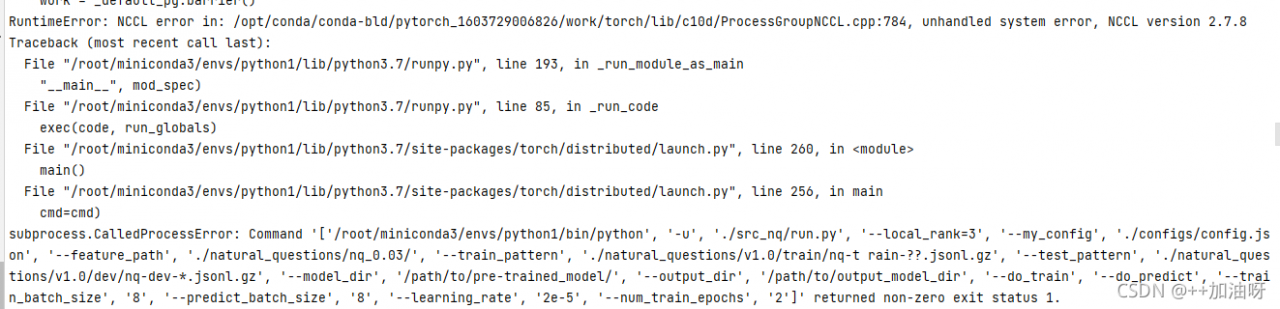Problem description

Perhaps parallel operation is not started???（

Solution:

(1) First, check the server GPU related information. Enter the pytorch terminal to enter the code

``````python
torch.cuda.is_available()# to see if cuda is available.
torch.cuda.device_count()# to see the number of gpu's.
torch.cuda.get_device_name(0)# to see the gpu name, the device index starts from 0 by default.
torch.cuda.current_device()# return the current device index.
``````

Ctrl+Z Exit
(2) cd enters the upper folder of the file to be run

`````` CUDA_VISIBLE_DEVICES=0,1,2,3,4,5 python -m torch.distributed.launch --nproc_per_node=6 #启动并行运算
``````

Plus files to run and related configurations

`````` CUDA_VISIBLE_DEVICES=0,1,2,3,4,5 python -m torch.distributed.launch --nproc_per_node=6  src_nq/create_examples.py --vocab_file ./bert-base-uncased-vocab.txt \--input_pattern "./natural_questions/v1.0/train/nq-train-*.jsonl.gz" \--output_dir ./natural_questions/nq_0.03/\--do_lower_case \--num_threads 24 --include_unknowns 0.03 --max_seq_length 512 --doc_stride 128
``````

Problem-solving!

# [Solved] AttributeError: ‘HTMLWriter‘ object has no attribute ‘_temp_names‘

Error Message (Error 1):

TypeError: render() got an unexpected keyword argument ‘mode‘

Solution for Error1:

Tried setting gym and pyglet to

• gym:0.17.1
• pyglet:1.5.0

Note: This method will solve the problem above.

However, An new error (Error 2) will be reported:

AttributeError: ‘HTMLWriter’ object has no attribute ‘_temp_names’

Solution for Error2:

• Open the .py file where you wrote your code (the same file you wrote your code in)
• Find your animate_frames method. (If you don’t have it, you can ignore it, I don’t have it, then just put the first block of code from step 3 at the top)
• Add the code before the animate_frames method (add the package to the top).
``````import matplotlib.pyplot as plt
from IPython.display import HTML

def display_animation(anim):
plt.close(anim._fig)
return HTML(anim.to_jshtml())``````

Find the following code:

``display(display_animation(anim, default_mode='XXX'))``

Change it to:

``display(display_animation(anim))``

The following code can be deleted or ignored:

``from JSAnimation.IPython_display import display_animation``

# [Solved] RuntimeError: a view of a leaf Variable that requires grad is being used in an in-place operation

source code

``````	def anim(i):
# update SMBLD
cur_beta_idx, cur_step = i // num_steps, i % num_steps
val = shape_range[cur_step]
mesh.multi_betas[0, cur_beta_idx] = val  # Update betas
fig.suptitle(f"{name.title()}\nS{cur_beta_idx} : {val:+.2f}", fontsize=50)  # update text

return dict(mesh=mesh.get_meshes(), equalize=False)

``````

Modified code

Add `with torch.no_grad():` will be OK!

``````	def anim(i):
# update SMBLD
cur_beta_idx, cur_step = i // num_steps, i % num_steps
val = shape_range[cur_step]
#print("\ncur_beta_idx:",cur_beta_idx,mesh.multi_betas[0, cur_beta_idx])
mesh.multi_betas[0, cur_beta_idx] = val  # Update betas
fig.suptitle(f"{name.title()}\nS{cur_beta_idx} : {val:+.2f}", fontsize=50)  # update text

return dict(mesh=mesh.get_meshes(), equalize=False)

``````

# [Solved] LeNet Script Train Error: AttributeError: ‘DictIterator’ object has no attribute ‘get_next’

My training environment:

Windows10 64bit；

MindSpore1.5.0-beta；

CPU；

python3.9；

When training Mnist data set with LeNet , the following error occurs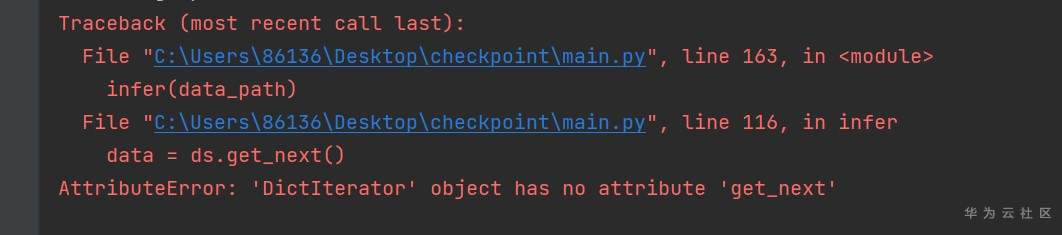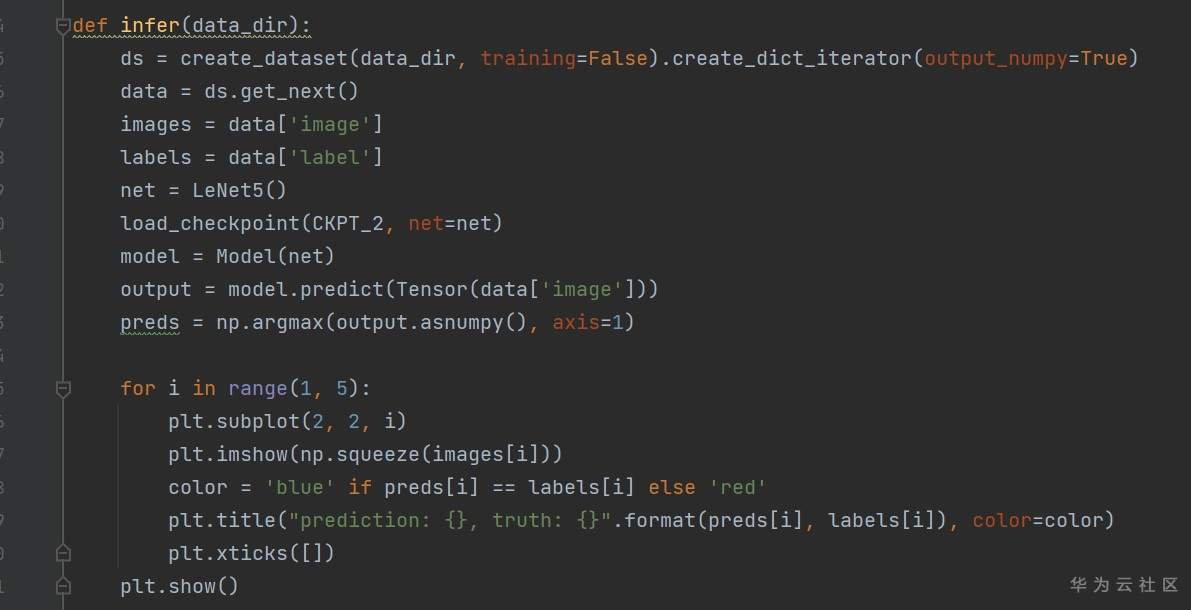How to solve this problem??

It’s true that the version is a bit old – the above use case needs to be modified if implemented on a newer version – from the current implementation of Iterator’s code, it no longer has the get_next method: https://gitee.com/mindspore/mindspore/blob/master/mindspore/python/mindspore/dataset/engine/iterators.py#L59

But it has __next__ method, so the above line can be modified, you can try it: > original: data = ds.get_next() > modified: data = next(ds)

# How to Solve wikiextractor Extract Wikipedia Corpus Error

When I extracted Wikipedia corpus, I first used the wikiextractor. Later, I found that it was always wrong, so it was useless. Since many people asked me how to extract the corpus, I now publish the code

I didn’t write the code, but I found it from a website. Because it took too long and I forgot the address of the website, I can’t post the original URL. If the author sees it, please send a private letter to my original URL

The author’s email address is: [email protected]

How to use: enter the command at the command line:

``python data_pre_process.py zhwiki-latest-pages-articles.xml.bz2(Wikipedia Corpus) wiki.zh.text(Saved files)``

Source code:

``````# -*- coding: utf-8 -*-
# Author: Pan Yang ([email protected])
# Copyrigh 2017
from __future__ import print_function

import logging
import os.path
import six
import sys

from IPython.core.page import page
from gensim.corpora import WikiCorpus

page.encoding = 'utf-8'

# Wrapping the Wikipedia xml corpus into txt format
# python data_pre_process.py zhwiki-latest-pages-articles.xml.bz2 wiki.zh.text
if __name__ == '__main__':
program = os.path.basename(sys.argv)
logger = logging.getLogger(program)

logging.basicConfig(format='%(asctime)s: %(levelname)s: %(message)s')
logging.root.setLevel(level=logging.INFO)
logger.info("running %s" % ' '.join(sys.argv))

# check and process input arguments
if len(sys.argv) != 3:
print("Using: python process_wiki.py enwiki.xxx.xml.bz2 wiki.en.text")
sys.exit(1)
inp, outp = sys.argv[1:3]
space = " "
i = 0

output = open(outp, 'w', encoding='utf-8')
wiki = WikiCorpus(inp, dictionary={})
for text in wiki.get_texts():
if six.PY3:
output.write(bytes(' '.join(text), 'utf-8').decode('utf-8') + '\n')
#   ###another method
#   output.write(space.join(map(lambda x: x.decode("utf-8"), str(text))) + '\n')
else:
output.write(space.join(text) + "\n")
i = i + 1
if i % 10000 == 0:
logger.info("Saved " + str(i) + " articles")

output.close()
logger.info("Finished Saved " + str(i) + " articles")``````

# [Solved] Error in Summary.factor ‘sum’ not meaningful for factors

Question:

The root cause is the wrong data type.

The factor type has no sum method

``````#create a vector of class vector
factor_vector <- as.factor(c(1, 7, 12, 14, 15))

#attempt to find min value in the vector
sum(factor_vector)

Error in Summary.factor(1:5, na.rm = FALSE) :
‘sum’ not meaningful for factors``````

Solution:
Convert to numeric values and use the as.numeric function.
mydata\$value<-as.numeric(mydata\$value)
is.numeric(mydata\$value)

``````#convert factor vector to numeric vector and find the min value
new_vector <- as.numeric(as.character(factor_vector))
sum(new_vector)

# 49``````

Complete error:

```#create a vector of class vector
factor_vector <- as.factor(c(1, 7, 12, 14, 15))

#attempt to find min value in the vector
sum(factor_vector)

Error in Summary.factor(1:5, na.rm = FALSE) :
‘sum’ not meaningful for factors```

Other (the minimum value can be obtained for numeric value, string and date type)

Numeric value, string and date type can all be maximized. Similarly, the maximum value can be obtained.

``````numeric_vector <- c(1, 2, 12, 14)
max(numeric_vector)

# 14

character_vector <- c("a", "b", "f")
max(character_vector)

# "f"

date_vector <- as.Date(c("2019-01-01", "2019-03-05", "2019-03-04"))
max(date_vector)

# "2019-03-05"``````

The R language is called R partly because of the names of the two R authors (Robert gentleman and Ross ihaka) and partly because of the influence of Bell Labs s language (called the dialect of s language).

R language is a mathematical programming language designed for mathematical researchers. It is mainly used for statistical analysis, drawing and data mining.

If you are a beginner of computer programs and are eager to understand the general programming of computers, R language is not an ideal choice. You can choose python, C or Java.

Both R language and C language are the research achievements of Bell Laboratories, but they have different emphasis areas. R language is an explanatory language for mathematical theory researchers, while C language is designed for computer software engineers.

R language is a language for interpretation and operation (different from the compilation and operation of C language). Its execution speed is much slower than that of C language, which is not conducive to optimization. However, it provides more abundant data structure operation at the syntax level and can easily output text and graphic information, so it is widely used in mathematics, especially in statistics

# [Solved] Error in Summary.factor ‘max’ not meaningful for factors

Question:

The root cause is the wrong data type.

The factor type has no max method

``````#create a vector of class vector
factor_vector <- as.factor(c(1, 7, 12, 14, 15))

#attempt to find max value in the vector
max(factor_vector)

#Error in Summary.factor(1:5, na.rm = FALSE) :
#  'max' not meaningful for factors``````

Solution:

Convert to numeric value or string, here convert to numeric value.

mydata\$value<-as.numeric(mydata\$value)
is.numeric(mydata\$value)

``````#convert factor vector to numeric vector and find the max value
new_vector <- as.numeric(as.character(factor_vector))
max(new_vector)

# 15``````

Full error:

```#create a vector of class vector
factor_vector <- as.factor(c(1, 7, 12, 14, 15))

#attempt to find max value in the vector
max(factor_vector)

#Error in Summary.factor(1:5, na.rm = FALSE) :
#  'max' not meaningful for factors```

Other (numeric value, string, date type can be the maximum value)

Numeric value, string and date type can all be the maximum value, and similarly, the minimum value can be obtained.

``````numeric_vector <- c(1, 2, 12, 14)
max(numeric_vector)

# 14

character_vector <- c("a", "b", "f")
max(character_vector)

# "f"

date_vector <- as.Date(c("2019-01-01", "2019-03-05", "2019-03-04"))
max(date_vector)

# "2019-03-05"``````

# [Solved] error indicates that your module has parameters that were not used in producing loss

Error Messages:
RuntimeError: Expected to have finished reduction in the prior iteration before starting a new one. This error indicates that your module has parameters that were not used in producing loss. You can enable unused parameter detection by passing the keyword argument `find_unused_parameters=True` to `torch.nn.parallel.DistributedDataParallel`, and by
making sure all `forward` function outputs participate in calculating loss.
If you already have done the above, then the distributed data parallel module wasn’t able to locate the output tensors in the return value of your module’s `forward` function. Please include the loss function and the structure of the return value of `forward` of your module when reporting this issue (e.g. list, dict, iterable).
Parameter indices which did not receive grad for rank 0: 160 161 182 183 204 205 230 231 252 253 274 275 330 331 414 415 438 439 462 463 486 487 512 513 536 537 560 561 584 585
In addition, you can set the environment variable TORCH_DISTRIBUTED_DEBUG to either INFO or DETAIL to print out information about which particular parameters did not receive gradient on this rank as part of this error

Solution:

Original Code

`````` class AttentionBlock(nn.Module):
def __init__(
self,
):
super().__init__()
self.encoder_kv = conv_nd(1, 512, channels * 2, 1) #这行没有注释掉
self.encoder_qkv = conv_nd(1, 512, channels * 3, 1)
self.trans = nn.Linear(resolution*resolution*9+128,resolution*resolution*9)
def forward(self, x, encoder_out=None):
b, c, *spatial = x.shape
x = x.reshape(b, c, -1)
qkv = self.qkv(self.norm(x))
if encoder_out is not None:
# encoder_out = self.encoder_kv(encoder_out)  #这行代码注释了，没有用self.encoder_kv
encoder_out = self.encoder_qkv(encoder_out)
return encode_out
``````

Error reason:

self.encoder_kv is written in def__init__, but not used in forward, resulting in an error in torch.nn.parallel.DistributedDataParallel. Correction method

Modified code

``````class AttentionBlock(nn.Module):
def __init__(
self,
):
super().__init__()
#self.encoder_kv = conv_nd(1, 512, channels * 2, 1) #这行在forward中没有用到注释掉
self.encoder_qkv = conv_nd(1, 512, channels * 3, 1)
self.trans = nn.Linear(resolution*resolution*9+128,resolution*resolution*9)
def forward(self, x, encoder_out=None):
b, c, *spatial = x.shape
x = x.reshape(b, c, -1)
qkv = self.qkv(self.norm(x))
if encoder_out is not None:
# encoder_out = self.encoder_kv(encoder_out)
encoder_out = self.encoder_qkv(encoder_out)
return encode_out
``````

Comment out the function self.encoder_kv = conv_nd(1, 512, channels * 2, 1) that is not used in the forward, and the program will run normally.

# MindSpore Error: [ERROR] MD:unexpected error.Not a valid index

ERROR:[MD]:unexpected error. Not a valid index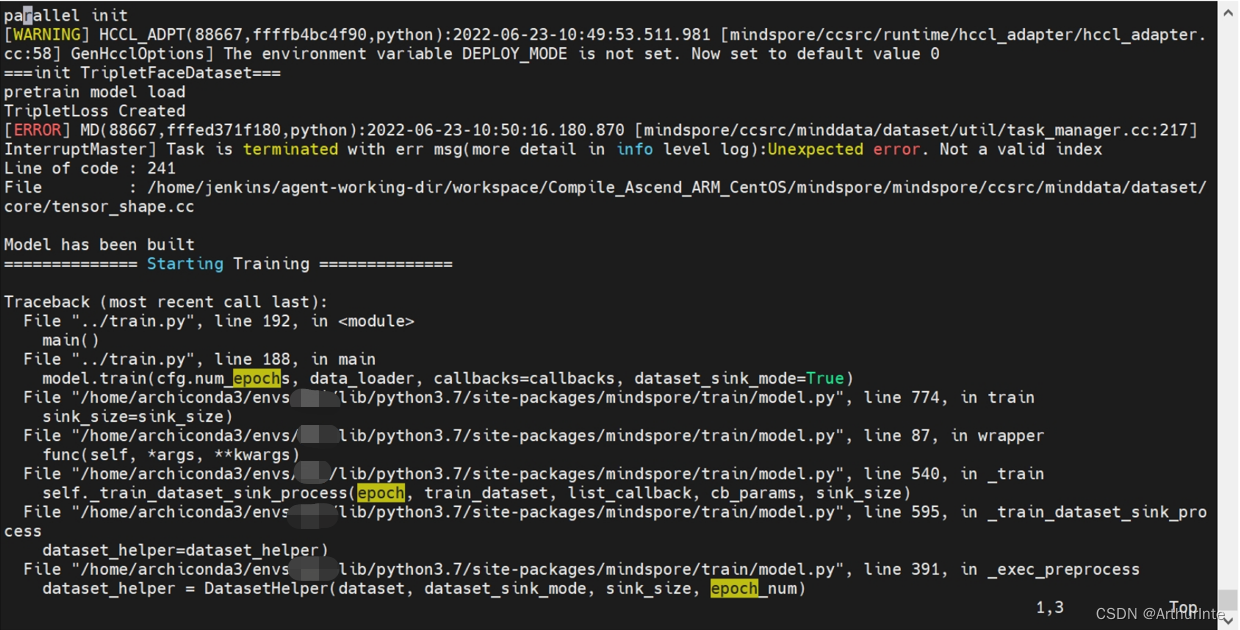problem phenomenon: a single card does not report an error, and the training process can be correctly executed. However, when switching to distributed training, an error in the diagram is reported. After troubleshooting, the cause of the error is found to be the wrong use of distributed sampling
the error reporting method is as follows: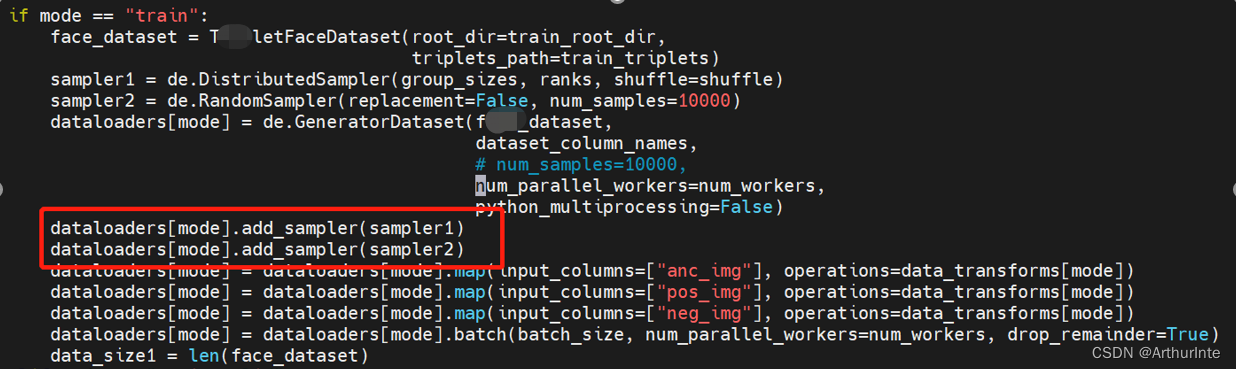the order of distributed sampling and random sampling needs to be changed. The correct way is to perform random sampling first and then distributed sampling

The correct modification is as follows: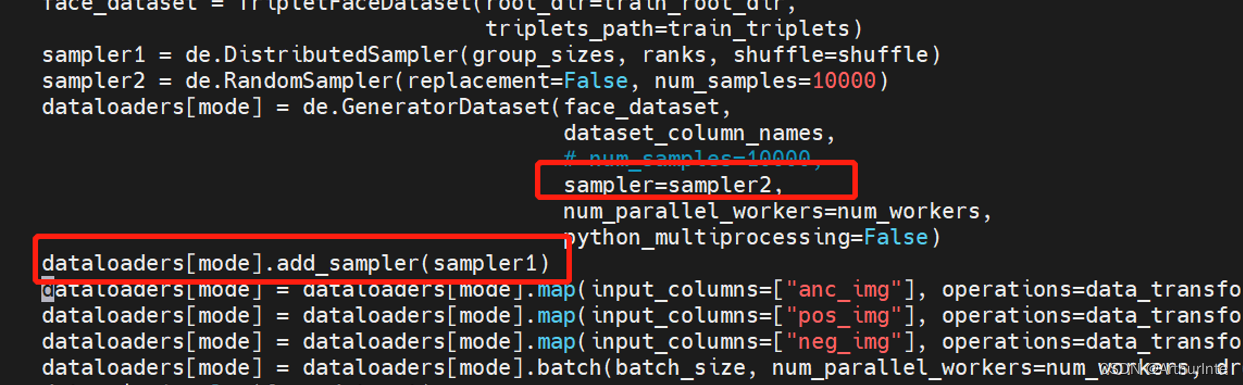distributed training can be performed correctly after modification

# [Solved] mindinsight modelart Error: RuntimeError: An attempt has been made to start a new process before…

Question:

Mindinsight uses error reporting on modelart.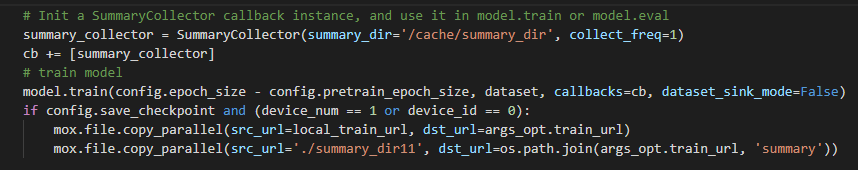After adding the summary and training some epoch normally, the operation will report an error: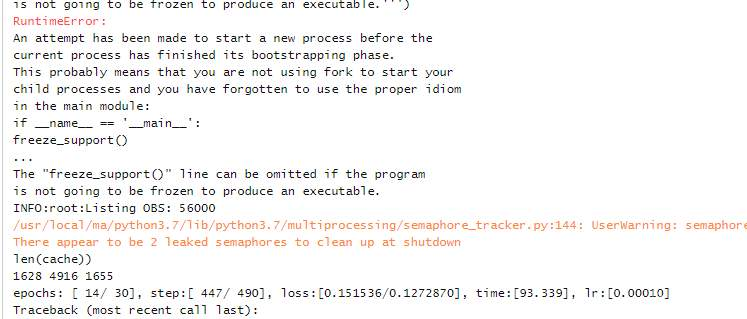Solution:

When using SummaryCollector, you need to put the code block in `if__name__ == __main__:`

The official mindspire tutorial has been updated. You can refer to the writing method of the latest tutorial: collect summary data – mindspire master document

Codes like this:

``````def train():
summary_collector = SummaryCollector(summary_dir='./summary_dir')

...

model.train(...., callback=[summary_collector])

if __name__ == '__main__':
train()``````

# [Solved] ValueError: Error when checking input: expected conv2d_input to have 4 dimensions

Error Messages:

ValueError: Error when checking input: expected conv2d_input to have 4 dimensions, but got array with shape (150, 150, 3)
Codes:

``````image = mpimg.imread("./ima/b.jpg")
image = image/255
classe = model.predict(image, batch_size=1)
``````

Reason:
the input format is incorrect
solution:
standardize the dataset

Specific solutions:

``````image = mpimg.imread("./ima/b.jpg")
image = image.reshape(image.shape(1,150,150,3)/255
classe = model.predict(image, batch_size=1)``````

# [Solved] Error: ‘attrition‘ is not an exported object from ‘namespace:rsample‘

Error: ‘attrition’ is not an exported object from ‘namespace:rsample’

# Import package and library

``````# load required packages
library(rsample)
library(dplyr)
library(h2o)
library(DALEX)

# initialize h2o session
h2o.no_progress()
h2o.init()
##  Connection successful!
##
## R is connected to the H2O cluster:
##     H2O cluster uptime:         4 hours 30 minutes
##     H2O cluster timezone:       America/New_York
##     H2O data parsing timezone:  UTC
##     H2O cluster version:        3.18.0.11
##     H2O cluster version age:    1 month and 17 days
##     H2O cluster total nodes:    1
##     H2O cluster total memory:   1.01 GB
##     H2O cluster total cores:    4
##     H2O cluster allowed cores:  4
##     H2O cluster healthy:        TRUE
##     H2O Connection ip:          localhost
##     H2O Connection port:        54321
##     H2O Connection proxy:       NA
##     H2O Internal Security:      FALSE
##     H2O API Extensions:         XGBoost, Algos, AutoML, Core V3, Core V4
##     R Version:                  R version 3.5.0 (2018-04-23)``````

#Data preprocessing and processing to h2o format;

Error: ‘attrition’ is not an exported object from ‘namespace:rsample’

``````#

# classification data
df <- rsample::attrition %>%
mutate_if(is.ordered, factor, ordered = FALSE) %>%
mutate(Attrition = recode(Attrition, "Yes" = "1", "No" = "0") %>% factor(levels = c("1", "0")))

# convert to h2o object
df.h2o <- as.h2o(df)

# create train, validation, and test splits
set.seed(123)
splits <- h2o.splitFrame(df.h2o, ratios = c(.7, .15), destination_frames = c("train","valid","test"))
names(splits) <- c("train","valid","test")

# variable names for resonse & features
y <- "Attrition"
x <- setdiff(names(df), y) ``````

Solution:

You can use the attrition dataset of DALEX package directly;

Remove resample:

``````#

# classification data
df <- attrition %>%
mutate_if(is.ordered, factor, ordered = FALSE) %>%
mutate(Attrition = recode(Attrition, "Yes" = "1", "No" = "0") %>% factor(levels = c("1", "0")))

# convert to h2o object
df.h2o <- as.h2o(df)

# create train, validation, and test splits
set.seed(123)
splits <- h2o.splitFrame(df.h2o, ratios = c(.7, .15), destination_frames = c("train","valid","test"))
names(splits) <- c("train","valid","test")

# variable names for resonse & features
y <- "Attrition"
x <- setdiff(names(df), y) ``````

Full Error Messages:

```> # classification data
> df <- rsample::attrition %>%
+     mutate_if(is.ordered, factor, ordered = FALSE) %>%
+     mutate(Attrition = recode(Attrition, "Yes" = "1", "No" = "0") %>% factor(levels = c("1", "0")))
Error: 'attrition' is not an exported object from 'namespace:rsample'
>```